# Test: Networks- 1

Test Description

## 15 Questions MCQ Test GATE Electrical Engineering (EE) 2023 Mock Test Series | Test: Networks- 1

Test: Networks- 1 for Electronics and Communication Engineering (ECE) 2023 is part of GATE Electrical Engineering (EE) 2023 Mock Test Series preparation. The Test: Networks- 1 questions and answers have been prepared according to the Electronics and Communication Engineering (ECE) exam syllabus.The Test: Networks- 1 MCQs are made for Electronics and Communication Engineering (ECE) 2023 Exam. Find important definitions, questions, notes, meanings, examples, exercises, MCQs and online tests for Test: Networks- 1 below.
Solutions of Test: Networks- 1 questions in English are available as part of our GATE Electrical Engineering (EE) 2023 Mock Test Series for Electronics and Communication Engineering (ECE) & Test: Networks- 1 solutions in Hindi for GATE Electrical Engineering (EE) 2023 Mock Test Series course. Download more important topics, notes, lectures and mock test series for Electronics and Communication Engineering (ECE) Exam by signing up for free. Attempt Test: Networks- 1 | 15 questions in 45 minutes | Mock test for Electronics and Communication Engineering (ECE) preparation | Free important questions MCQ to study GATE Electrical Engineering (EE) 2023 Mock Test Series for Electronics and Communication Engineering (ECE) Exam | Download free PDF with solutions
 1 Crore+ students have signed up on EduRev. Have you?
Test: Networks- 1 - Question 1

###If each branch of a 'Y' circuit has impedance equal tom , then each branch of the equivalent delta circuit has impedance equal to

Detailed Solution for Test: Networks- 1 - Question 1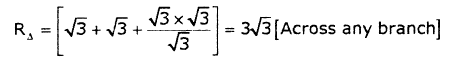Test: Networks- 1 - Question 2

### The transmission parameters for circuit shown below are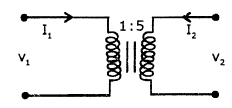Detailed Solution for Test: Networks- 1 - Question 2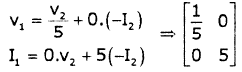Test: Networks- 1 - Question 3

### The rise time of the RC circuit shown below is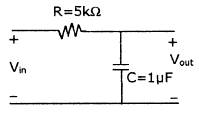Test: Networks- 1 - Question 4

A station in a network forwards incoming packets by placing them on its shortest output queue. What routing algorithm is being used?

Test: Networks- 1 - Question 5

A tree of the graph shown below is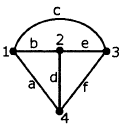Detailed Solution for Test: Networks- 1 - Question 5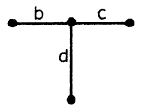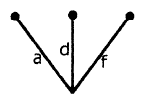Test: Networks- 1 - Question 6

The driving point function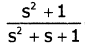can be realized as a

Test: Networks- 1 - Question 7

The equivalent resistance between A and B in the following network is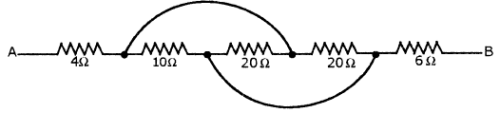Detailed Solution for Test: Networks- 1 - Question 7

The equivalent network is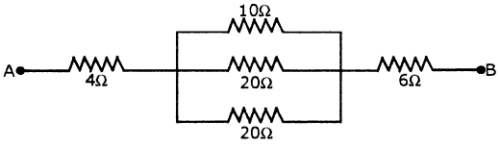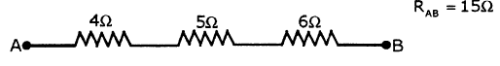Test: Networks- 1 - Question 8

The network shown below is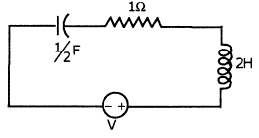Detailed Solution for Test: Networks- 1 - Question 8
1. Roots are complex conjugates
Test: Networks- 1 - Question 9

The impendance matrix for the circuit shown below is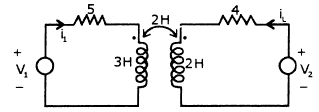Test: Networks- 1 - Question 10

The steady state voltage across the capacitors C1, C2 and C3 shown in the circuit below are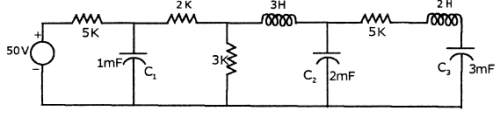Detailed Solution for Test: Networks- 1 - Question 10

Voltage across C1 under steady state =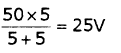Voltage across C2 is voltage across 31d/ resistance =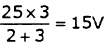voltage across C3 is equal to voltage across C2 under steady state = 15V

Test: Networks- 1 - Question 11

Which of the following is correct?

1. Cut set is the number of branches that are to be removed to break the graph into two parts.

2. Rank of cut set matrix = Rank of incidence matrix

Adding one twig to the existing co-tree results in one tie-set.

Detailed Solution for Test: Networks- 1 - Question 11

Cut set is minimum number of branches to be removed to break graph into two parts.

Test: Networks- 1 - Question 12

Find the voltage Vx in the given[/expand] circuit.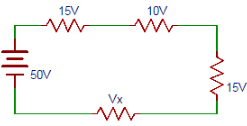Detailed Solution for Test: Networks- 1 - Question 12

From the circuit applying Kirchhoff’s voltage law, we can write 50= 15+ 10+ 15+Vx => Vx= 10V.

Test: Networks- 1 - Question 13

The Dual of the network shown below is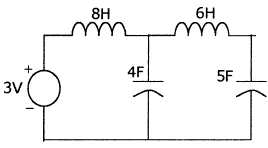Test: Networks- 1 - Question 14

Calculate the power dissipated in 3.Ω resistor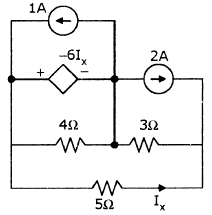Test: Networks- 1 - Question 15

In the circuit shown below, Vs = 250 sin 500t,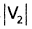= 200. If R = 100Ω, then the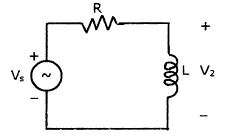Detailed Solution for Test: Networks- 1 - Question 15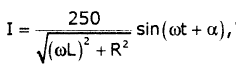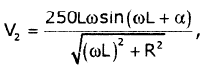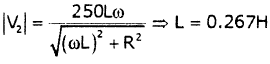## GATE Electrical Engineering (EE) 2023 Mock Test Series

22 docs|274 tests
Information about Test: Networks- 1 Page
In this test you can find the Exam questions for Test: Networks- 1 solved & explained in the simplest way possible. Besides giving Questions and answers for Test: Networks- 1, EduRev gives you an ample number of Online tests for practice

## GATE Electrical Engineering (EE) 2023 Mock Test Series

22 docs|274 tests(Scan QR code)